## Posts

Showing posts from 2022

### Happy New Year!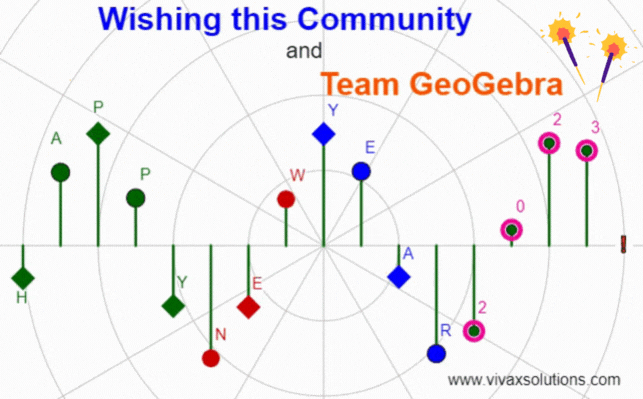### Differentiation: problem solving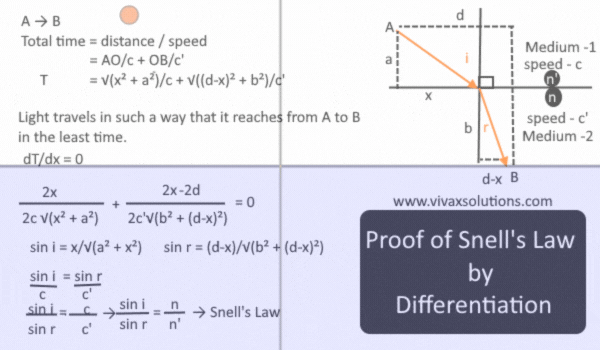Snell's Law When light refracts from one medium to another, the ratio of sine of the angles is equal to the ratio of refractive index of each corresponding medium. Snell's Law implies that light, when refracting from one medium to another, takes the shortest path. We can show by differentiation that when the light takes the shortest path, it obeys Snell's Law, verifying the 400-year-old law discovered  by the Dutch astronomer and mathematician.

### Pascal's Triangle in Python for Computer Science: GCSE, IGCSE and A Level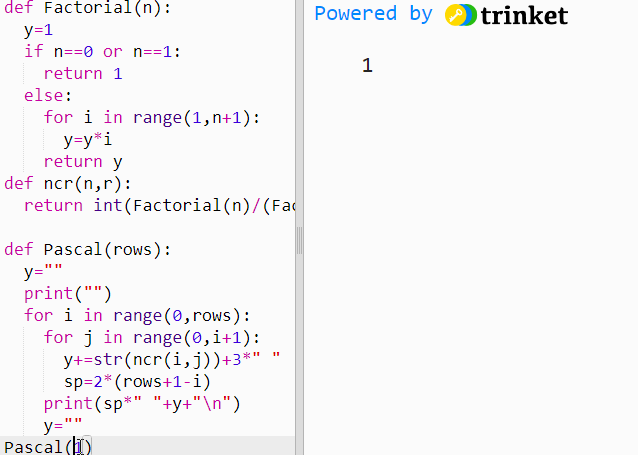The above simulation is produced in Python with aid of three functions. They are as follows: Factorial function  A function to generate combinations - nCr A function to produce the Pascal's Triangle You can practise it interactively with the following simulation. Please make sure you keep the number of rows below 10 so that the format of the output stays the way it should. The number of rows is the only parameter that Pascal(n) function needs.

### Straight Line Graph Generator for Maths Teachers: GCSE, IGCSE, GCE O Level, GCE A Level, A Level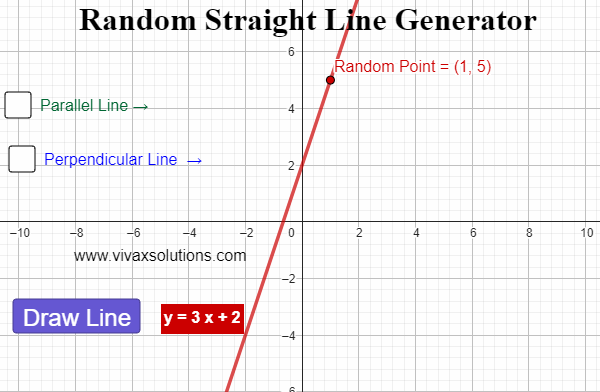Created with GeoGebra, this applet lets you generate straight line graphs at random, displaying the corresponding equation for each. In addition, you can find a parallel line - and its equation - for each line. Moreover, for each generated line, a random point is created. Then, the perpendicular line  that goes through the point in question can be created too. It is ideal for teachers at GCSE, IGCE, GCE O Level, GCE A Level and of course, A Level. The interactive simulation of the above is as follows: you can generate unlimited number of lines for practice for you students: just press the button - Draw Line -  to create random lines.

### Merry Christmas to all!Created with GeoGebra, the simulation shows how the potential energy of the Santa and the team, PE,  varies with the changing height of its their magical path to the ultimate destination.

### Creating Pascal's Triangle in C#: for computer science at GCSE, IGCE, A Level and programmers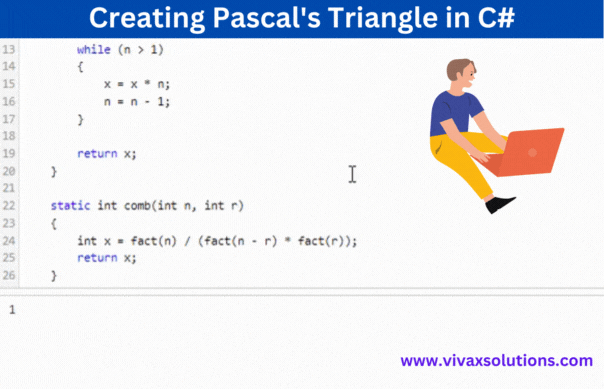Creation of Pascal's Triangle in C# The above animation is produced in C# programming language for students who do computer science at GCSE, IGCSE, IB and A Level. The code snippet consists of four major function that collectively produce the Pascal's Triangle. They are: A factorial function A function to generate combinations A function to generate Pascal's Triangle in the correct format The main function to run the function in step 3). The code snippet is given below; you can practise it interactively by providing the function, Pascal's Triangle(n) , the parameter required - the number of rows. Since the formatting is programmed up to 10 rows, please use a number below 10.

### Straight Line Graph Generator: for Year 9, GCSE Maths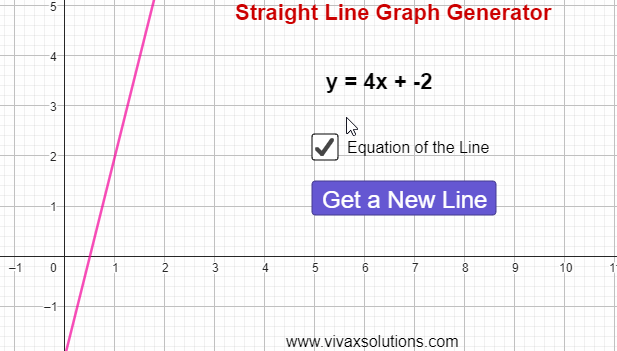The equation of a straight line is given by the formula, y = mx + c, where m and c are the gradient and intercept respectively. The gradient, m, is the slope or steepness of the line; the intercept, c, is the point where it crosses the y-axis at. E.g. A straight line passes through (1,2) and (3, 8). Find its equation. m = (8-2)/(3-1) = 3 m = 3 x = 1 y = 2 y = mx + c 2 = 3(1) + c c =  -1 The equation of the straight line is y = 3x - 1. Using the following simulation, you can create lines at random, calculate the equation as above and then check the answer, in order to be highly competent in the topic.

### Constructions for Maths: GCSE, IGCSE and GCE - OL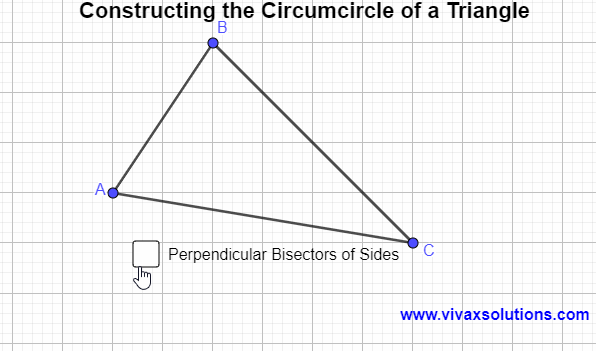A circumcircle is a circle that touches the three vertices of a circle from outside. It can easily be constructed by the following steps: Construct the perpendicular bisectors of each side of the triangle Locate the point of intersection of the bisectors The point of intersection is the centre of the circumcircle Construct the circle with centre in step 3). It is the circumcircle of the triangle in question.

### Correlation and the Line of Best Fit - for GCSE, IGCSE and A-Level Maths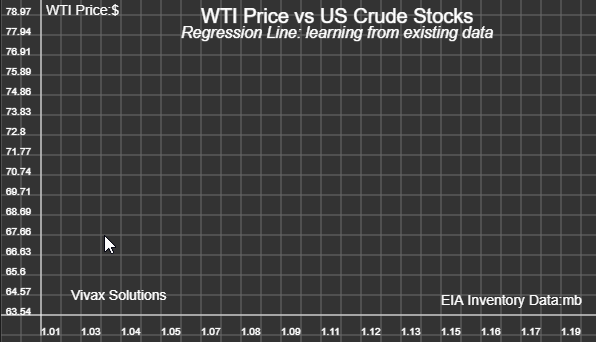The above animation shows how a code snippet written in JavaScript determines the line of best fit for an evolving set of data. The line is drawn accurately while performing the mathematical requirements for it to be accurate and minimizing the known errors. This is a stepping stone to AI - Artificial Intelligence - that can be best described as the ML - machine learning. In this case, the machine is learning from its own experience in plotting the dots on the grid. All you have to do is clicking at random points on the grid; the sketching the line of best is the responsibility of the programme. You can practise and have fun on your own here: A mild introduction to Machine Learning

### Solving equations by iteration - GCSE, IGCSE and A Level maths - using Python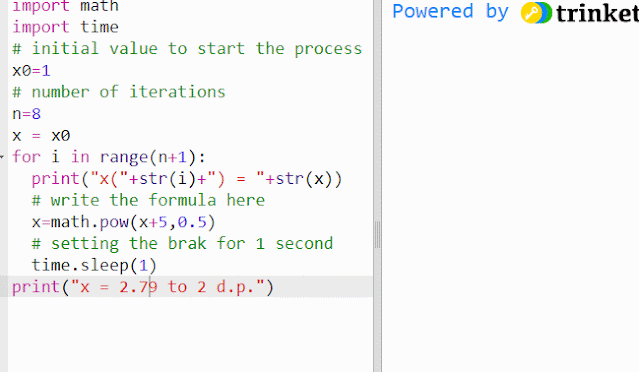Iteration If f(x) =0, it can be rearranged in the form of x = g(x), where both f(x) and g(x) are functions of x. Then x = g(x) is said to be in iterative form. E.g. x² - x - 5 = 0 x² = x + 5 x n+1 = √(x n + 5) - f(x) = 0 in iterative form If x n is known, x n+1 can be calculated. The above is not the only iterative form, though: two more iterative forms from can be derived from it; every form, however, may not produce the required result! it's important to choose the correct form. In order to start the process, the initial value, x 0 , is usually given. When the values of x becomes almost constant, the last value can be approximated as the solution or the root of the equation, to an appropriate significant level. For example, the above simulation shows how the process can be triggered off by taking x 0 = 1.  You can practise it here interactively; just change the value of x 0 and run the simulation:

### How to measure atmospheric pressure by a mercury barometer - a toxic legacy!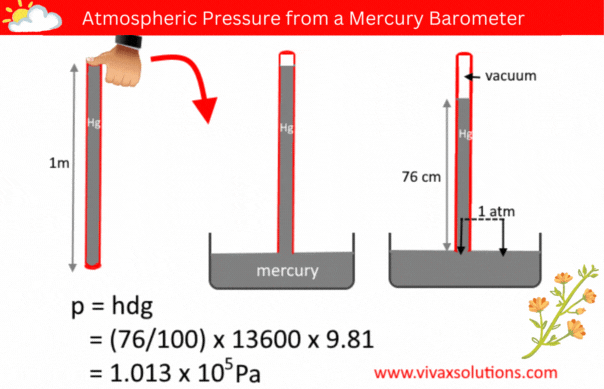The pressure of a liquid column is given by the formula, p = hdg, where h, d and g are the depth/height, density and the acceleration due to gravity respectively.  A few decades ago, in order to measure the atmospheric pressure, a simple barometer was used. It consisted of a thin, long, glass tube - sealed at one end - a laboratory stand with a clamp to hold it and a container full of mercury. The glass tube was usually a meter in length. In a school lab, a technician normally used to fill it with mercury to the brim, place his thumb at the open end, before inverting it and placed in the mercury container, while holding the tube vertically with the aid of the stand and clamp.   When left for a while for mercury to settle down, the technician measure the height of the mercury column. If the location in question is at a level closer to the sea level, the height of the mercury column is usually 76 cm. Since the pressure of a liquid at the same level is equal, the above diagram shows that

### Differentiating Trigonometric Functions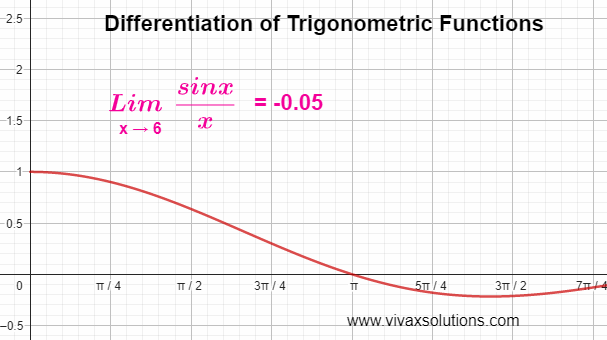When it comes to differentiating the functions of sin x and cos x, we need to use two fractions of the functions in question, when the variable approaches zero must be considered. They are as follows: lim sin (x) / x, as x → 0 lim (cos x - 1) / x as x → 0 Based on the above, sin x and cos x can be differentiated as follows: y = sin x → dy/dx = cos x y = cos x → dy/dx = - sin x y = tan x  → dy/dx = sec² x Differentiation of sine function

### Verification of the area of a circle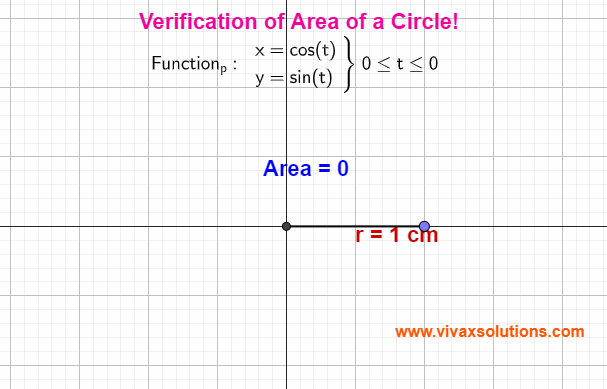Area of a circle by parametric equations The proof with the aid of parametric equation is as follows:

### Energy changes in simple harmonic motion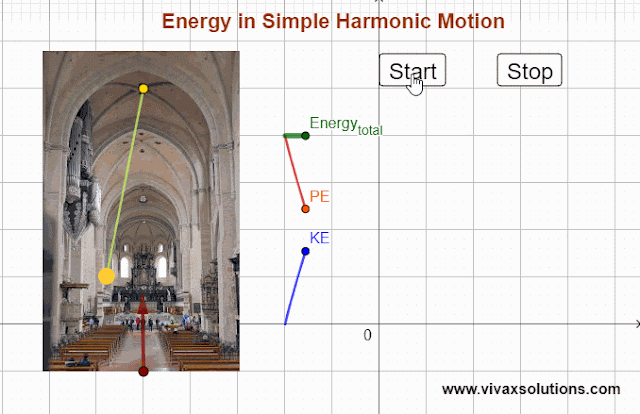The above simulation of the simple harmonic motion involving a pendulum shows how the potential energy, PE and kinetic energy, KE, change with the position of the pendulum, provided that the latter swings through a small angle. As you can see the total energy of the system remains the same: the loss of KE turns into PE and vice versa. In order to practise this interactively, along with a few related simulations under the SHM, please follow the link below: A comprehensive and interactive tutorial on the SHM is here .

### Simple Harmonic Motion of Pendulum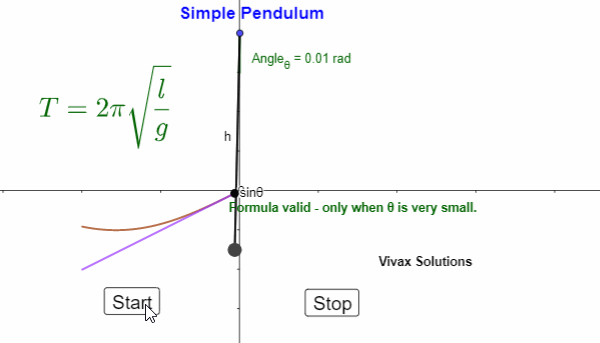The above simulation shows how the angle of oscillation determines the validity of the formula of the simple pendulum. During the process of deriving the formula, we assume the angle is small and measured in radians.  For the full tutorial, please follow this link .

### A Level further maths: equation of a half line - complex numbers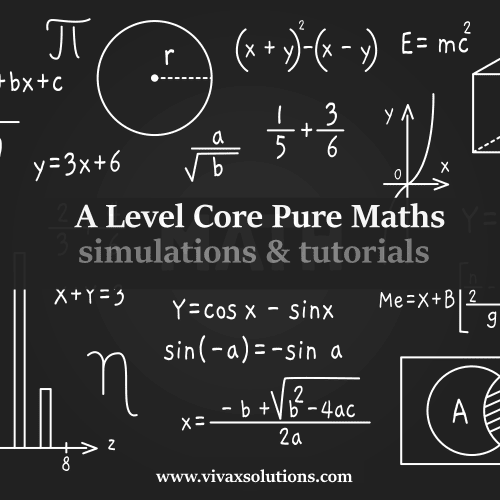The following simulation, which is fully interactive, shows you how to  derive the equation of a half line . A half line , by definition,  is a line that stretches from a point in one direction up to infinity.  You may change the position of the arbitrary point, denoted by Z, and the equation of the half-line will be automatically derived.  🎕 I am immensely thankful to the GeoGebra foundation for providing the programmers with this platform to play with amazing tools to  learn, share and above all, have fun - at the same time.

### Locus of a point: A Level further maths - complex numbersWith the following simulation, you can find out the equation of the locus of a point that moves in such a way that Argument(Z - z1) = Θ. The locus of the point is a half line - a line that starts from a point and moves only in one direction from it up to infinity.  You can change the position of Z - along with the argument - and find the equation of the half -line.

### Resultant Moment of Forces - GCSE, IGCSE and A Level physics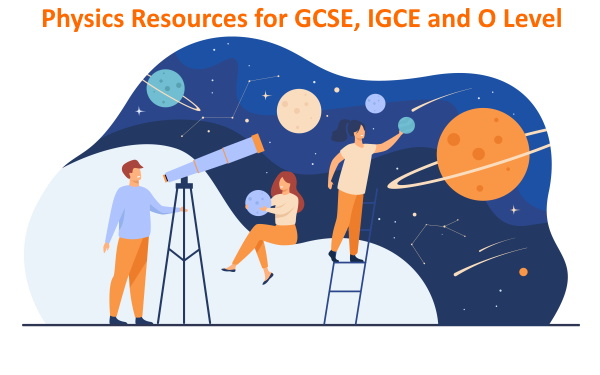With the following simulation, you can practise the moment of forces interactively. Moment of a force = force X perpendicular distance from the pivot The resultant moment or the total moment is the algebraic sum of the moments  of forces.  In the simulation, the clockwise moment is considered as positive. To increase the magnitude of a force, drag the end of each force/s up or down. The force that is closest to A can even be moved diagonally. Move the forces and study the way the total or resultant moment changes.

### A Level Core Maths: complex numbers and lociThe above simulation can be used to learn the locus of a point, under given conditions. Just move the point, Z, using the mouse and watch the appearance and disappearance of the shaded region. Alternatively, you can use the play button at the bottom-right corner too.

### Multiple Choice Questions on Capacitors for A Level PhysicsMultiple Choice Questions - A Level Physics - Capacitors - Time: 40 minutes Created and Programmed by Vivax Solutions   Start the Quiz   Submit Quiz

### Quiz: Multiple Choice Questions in Python for Computer Science - GCSE, iGCSE, O Level and A LevelMultiple Choice Questions in Python - for GCSE, IGCSE & A -Level - Time: 15 minutes Created and Programmed  by Vivax Solutions   The quiz of multiple choice questions is aimed at the students at GCSE, IGCSE, O Level and A Level in order to check their basic programming knowledge in Python. The quiz is evolving: at the beginning, there will be 20 questions, timed for 15 minutes. I will keep adding questions in due course so that aspiring users / programmers can make progress while play with them. Please watch this space! Start the Quiz   Submit Quiz